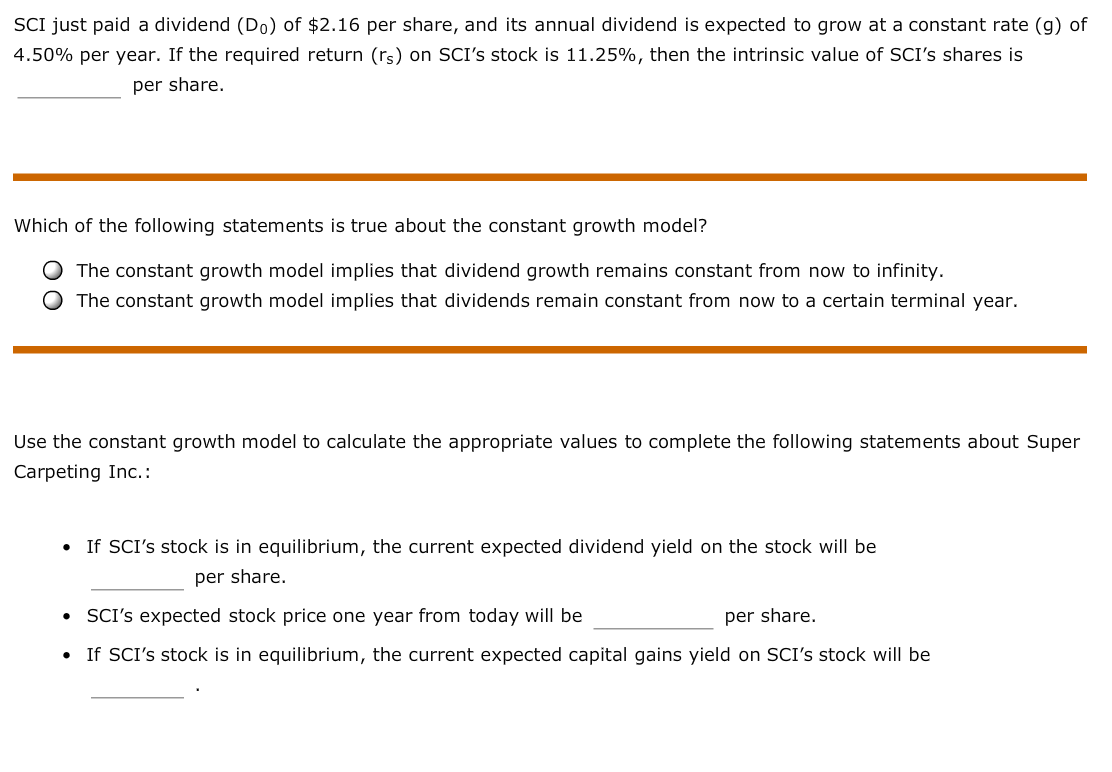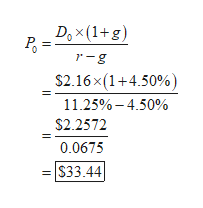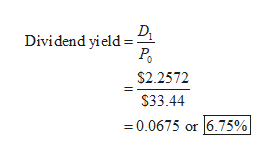SCI just paid a dividend (Do) of \$2.16 per share, and its annual dividend is expected to grow at a constant rate (g) of4.50% per year. If the required return (rs) on SCI's stock is 11.25%, then the intrinsic value of SCI's shares isper share.Which of the following statements is true about the constant growth model?The constant growth model implies that dividend growth remains constant from now to infinity.The constant growth model implies that dividends remain constant from now to a certain terminal year.Use the constant growth model to calculate the appropriate values to complete the following statements about SuperCarpeting Inc.:If SCI's stock is in equilibrium, the current expected dividend yield on the stock will beper shareSCI's expected stock price one year from today will beper shareIf SCI's stock is in equilibrium, the current expected capital gains yield on SCI's stock will be

Questionhelp_outlineImage TranscriptioncloseSCI just paid a dividend (Do) of \$2.16 per share, and its annual dividend is expected to grow at a constant rate (g) of 4.50% per year. If the required return (rs) on SCI's stock is 11.25%, then the intrinsic value of SCI's shares is per share. Which of the following statements is true about the constant growth model? The constant growth model implies that dividend growth remains constant from now to infinity. The constant growth model implies that dividends remain constant from now to a certain terminal year. Use the constant growth model to calculate the appropriate values to complete the following statements about Super Carpeting Inc.: If SCI's stock is in equilibrium, the current expected dividend yield on the stock will be per share SCI's expected stock price one year from today will be per share If SCI's stock is in equilibrium, the current expected capital gains yield on SCI's stock will be fullscreen
Step 1

Calculate the intrinsic value per share as follows:help_outlineImage TranscriptioncloseDox (1+g) r-g \$2.16x(1+4.50%) = 11.25%-4.50% \$2.2572 0.0675 \$33.44 fullscreen
Step 2

The statement “The constant growth model implies that dividends growth rate remains constant from now to infinityis true.

Step 3

Calculate the expected divid...help_outlineImage TranscriptioncloseDividend yield \$2.2572 \$33.44 0.0675 or 6.75% fullscreen

Want to see the full answer?

See Solution

Want to see this answer and more?

Our solutions are written by experts, many with advanced degrees, and available 24/7

See Solution
Tagged in